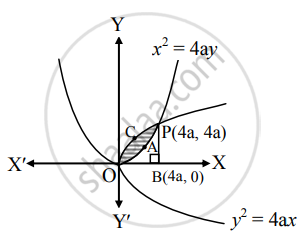# Find the area of the region bounded by the curves y2 = 4ax and x2 = 4ay - Mathematics and Statistics

Sum

Find the area of the region bounded by the curves y2 = 4ax and x2 = 4ay

#### SolutionGiven equations of the parabolas are

y2 = 4ax     .......(i)

and x2 = 4ay

∴ y = x^2/(4"a")   .......(ii)

From (i), we get

y2 = 4ax

∴ y = 2sqrt("a")sqrt(x)   ......(iii) ......[∵ In first quadrant, y > 0]

Find the points of intersection of y2 = 4ax and x2 = 4ay.

Substituting (ii) in (i), we get

((x^2)/(4"a")) = 4ax

∴ x4 = 64a3x

∴ x(x3 − 64a3) = 0

∴ x[x3 − (4a)3] = 0

∴ x = 0 and x = 4a

When x = 0, y = 0 and when x = 4a, y = 4a

∴ The points of intersection are O (0, 0) and P (4a, 4a).

Draw PB ⊥ OX.

Required area = area of the region OAPCO

= area of the region OBPCO – area of the region OBPAO

= area under the parabola y2 = 4ax – area under the parabola x2 = 4ay

= int_0^(4"a") 2sqrt("a")sqrt(x)  "d"x - int_0^(4"a")  x^2/(4"a")  "d"x     ......[From (iii) and (ii)]

= 2sqrt("a") int_0^(4"a")  x^(1/2)  "d"x - int_0^(4"a")  x^2/(4"a")  "d"x

= 2sqrt("a") [(x^(3/2))/(3/2)]_0^(4"a") - 1/(4"a") [x^3/3]_0^(4"a")

= 4/3 sqrt("a")[(4"a")^(3/2) - 0] - 1/(12"a") [(4"a")^3 - 0]

= 32/3 "a"^2 - 16/3 "a"^2

= 16/3 "a"^2 sq.units

Concept: Area Bounded by the Curve, Axis and Line
Is there an error in this question or solution?